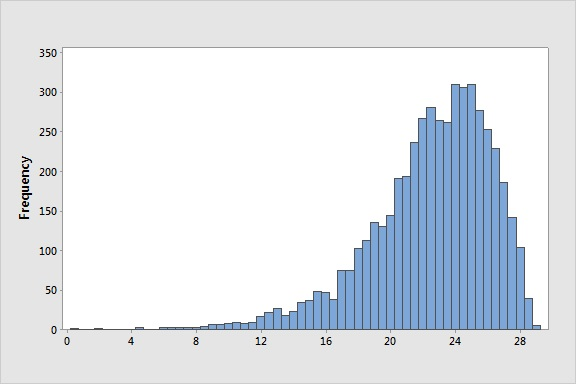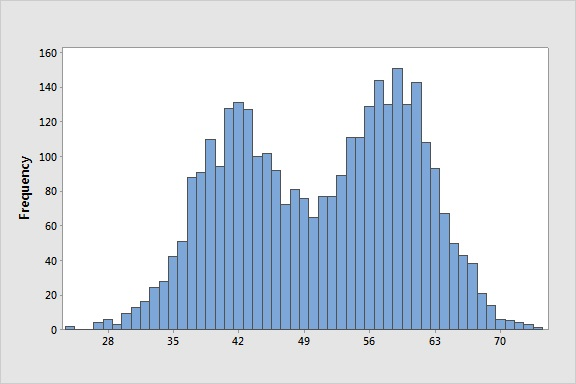﻿ Common shapes of distributions - MathBootCamps

# Common shapes of distributions

When making or reading a histogram, there are certain common patterns that show up often enough to be given special names. Sometimes you will see this pattern called simply the shape of the histogram or as the shape of the distribution (referring to the data set). While the same shape/pattern can be seen in many plots such as a boxplot or stemplot, it is often easiest to see with a histogram. In the examples below, we will look at each of these shapes and some of their important properties.

## Bell shaped / symmetric

Histograms that are bell shaped/symmetric appear to have one clear center that much of the data clusters around. As you get away from this center, there are fewer and fewer values.In the histogram above, that center is about 10. Notice that the tallest bars are around this value. The height of the bars is the frequency, or number of data values in a class. For values much smaller or larger than 10, there aren’t nearly as many data values.

This shape comes up frequently in every day life. For example weights and heights (when you look at genders individually) often follow this pattern. Most people are within a certain amount of the typical value with few extremes in either direction.

## Left skewed

In distributions that are skewed left, most of the data is clustered around a larger value, and as you get to smaller values, there are fewer and fewer seen in the data set. In the picture, there is essentially a tail going out to the left. You can see this in the histogram below where much of the data (the higher frequency) is around 24 or so. As you move to smaller numbers, there is less and less frequency. This means there are fewer and fewer observations.An easy to think about example of data which would have a skewed left distribution is scores on an easy test. Most students would do well, and as you get to lower scores, there would be fewer and fewer students with those scores.

## Right Skewed

Just like you saw with a left skewed distribution, distributions that are skewed right have a tail – but this time it is off to the right. This means that the data is generally clustered around a small value and as you look for larger and larger values, there are fewer and fewer.Looking at the histogram above, we can see most of the data is centered around 7 or so and that there are fewer and fewer larger data values. If test scores were skewed right it would not be a good thing! It would mean most students did poorly while only a few did well!

## Bimodal

You can think of a histogram with a bimodal shape as having two peaks. Instead of one clear center where there is are a lot of observations, there are two. Often this means that you are looking at two different groups and should take a closer look to see if you can separate them.In the example shown above, there is a peak around 42 or so and a peak around 58 or so. It is almost as if two symmetric/bell shaped histograms were shoved together. In real life, you might see this if you look at a data set for heights of people and it included both men and women. There would be a peak around the typical height of a man and a peak around the typical height of a women.

## Uniform

Data that follows a uniform pattern has approximately the same number of values in each group or class (represented by a bar).The histogram above follows a very uniform pattern as every bar is almost exactly the same height. This type of pattern shows up in some types of probability experiments. For example, if you were to take a 6 sided fair die and roll it many times (as in 100+) you would get a pattern that is approximately uniform.

You will find that the shape of a distribution is important in understanding the data set and in choosing the best measure of center, such as the mean or the median, to represent the data. This is why one of the first steps of analyzing a data set is to always plot your data!